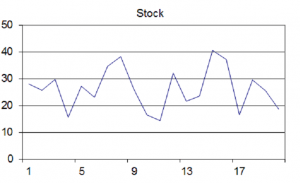8
Aug 16

## The pearls of AP Statistics 15Without using more Math, is it possible to drill deeper? Increase aggregation.

They say: Exercises 2.9, 2.11 compare a bar chart to a pie chart, Exercise 2.12 looks at the bar graph vs a Pareto chart and a dot plot vs a stem-and-leaf plot, Exercises 2.16, 20.18 prompt the reader to apply both the dot plot and a histogram. Exercise 2.21 tries to convince the reader that in some situations the stem-and-leaf plot is better than either the dot plot or histogram. Exercise 2.22 calls for the same comparison. Exercise 2.24 says: Repeat the preceding exercise for ...(bla-bla-bla) (Everywhere the references are to Agresti and Franklin).

I say: Championships in mental calculation are held in the categories: addition, multiplication, extracting roots and calendar calculation. No doubt, the world champions achieve outstanding results, but is any of them an outstanding mathematician? Arithmetic is a boundless ocean of elementary information. It is possible to demonstrate wonders at this level but never make contributions Ramanujan did (he was also a good calculator).

Shallow questions + Too many repetitions = Boredom

Your word is gold, use it sparingly. Students treat a simple exercise as another disturbing sound from the street traffic. Without using more Math, it's possible to raise the level by increasing aggregation. Ask deeper questions about several different notions in the same problem, forcing the students to correlate. Here is an example:Figure 1

Can you approximately indicate the mean of the variable in a histrogram (Figure 1)?Figure 2

If that figure were a Pareto diagram of type Figure 2, would you be able to indicate the mean?Figure 3

Where would you place approximately the mean on a time series plot in Figure 3?